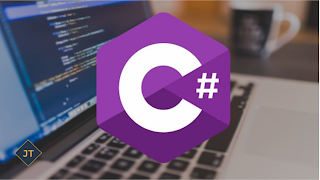Convert Decimal to Binary in C#

In this article I will explain how you will convert decimal number to binary in c#. So let's first we need understand what is decimal and what is binary number.

### Decimal Number

Decimal number is a base 10 number because it ranges from 0 to 9, there are total 10 digits between 0 to 9. Any combination of digits is decimal number such as 223, 585, 192, 0, 7 etc.

### Binary Number

Binary number is a base 2 number because it is either 0 or 1. Any combination of 0 and 1 is binary number such as 1001, 101, 11111, 101010 etc.

Before starting the Example Please remember the algorithm for the conversion.

#### Divide the number by 2 through % (modulus operator) and store the remainder in array Divide the number by 2 through / (division operator) Repeat the step 2 until the number is greater than zero

Let's take an Example to see how to convert Decimal number to Binary in C#.
```using System;
public class DecimalToBinary
{
public static void Main(string[] args)
{
int  n, i;
int[] a = new int;
Console.Write("Enter the number to convert: ");
for(i=0; n>0; i++)
{
a[i]=n%2;
n= n/2;
}
Console.Write("Binary of the given number= ");
for(i=i-1 ;i>=0 ;i--)
{
Console.Write(a[i]);
}
}
}  ```
Output:
```Enter the number to convert:10
Binary of the given number= 1010 ```

Program Explanation
This C# program, we are declaring the variable ‘n’ and ‘i’ Integer type. Using for loop check the value of ‘n’ variable is greater than 0 and also greater than 1.
The variable bin will get the value of the operation, the variable n value % 2; the Mod is used to obtain a division residue. Divide the numbers to indicate next to the symbol %, this case the number 2, because 2 is the binary numbers base. Then after obtaining the first residue the variable ‘n’ will get its own value between 2.